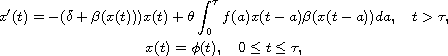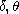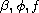Electron. J. Diff. Equ., Vol. 2014 (2014), No. 177, pp. 1-13.

### Global attractivity for nonlinear differential equations with a nonlocal term Boumediene Abdellaoui, Tarik Mohamed Touaoula

Abstract:whereare positive constants, andare positives continuous functions. The main results obtained in this paper are the following: (1) Using the Laplace transform, we prove the global asymptotic stability of the trivial steady state. (2) Under some additional hypotheses on the data and by constructing a Lyapunov functional, we show the asymptotic stability of the positive steady state. We conclude by applying our results to mathematical models of hematopoieses and Nicholson's blowflies.Boumediene Abdellaoui Laboratoire d'Analyse Nonlinéaire et Mathématiques Appliquées Département de Mathématiques Université Abou Bakr Belkaid Tlemcen, Tlemcen 13000, Algeria email: boumediene.abdellaoui@uam.es Tarik Mohamed Touaoula Laboratoire d'Analyse Nonlinéaire et Mathématiques Appliquées Département de Mathématiques Université Abou Bakr Belkaid Tlemcen, Tlemcen 13000, Algeria email: touaoula_tarik@yahoo.fr, tarik.touaoula@univ-tlemcen.dz